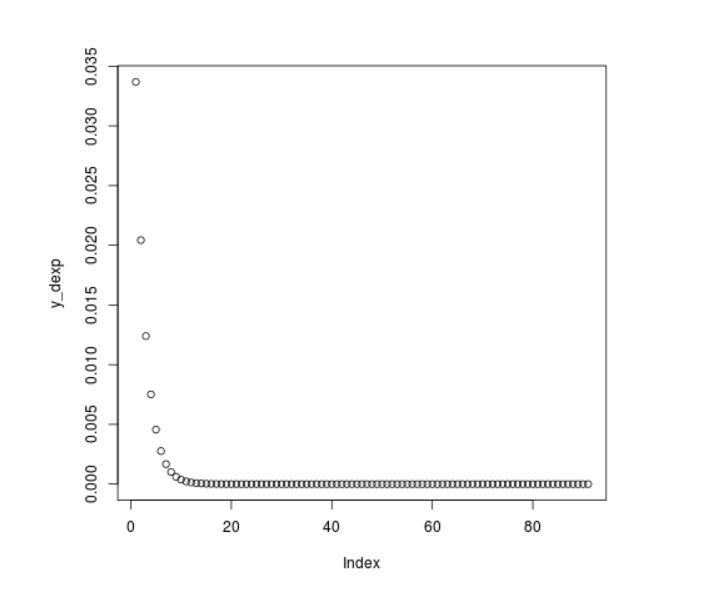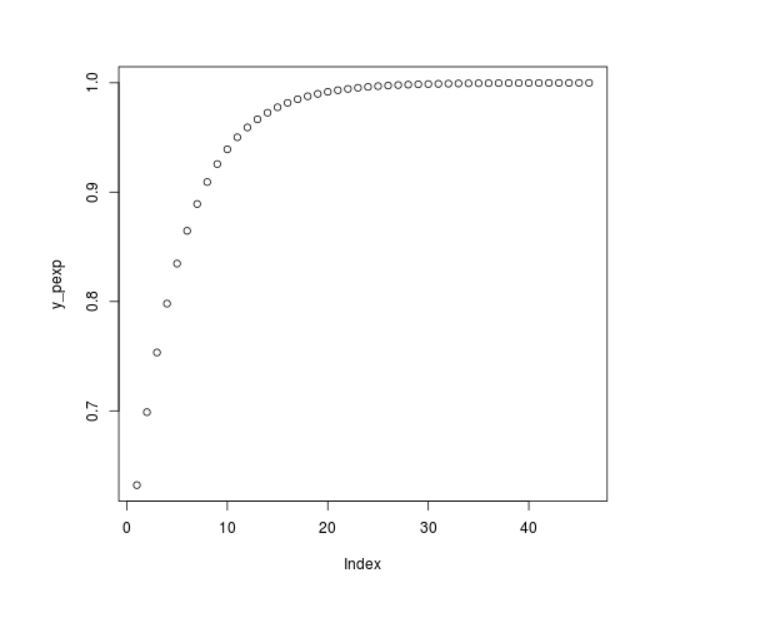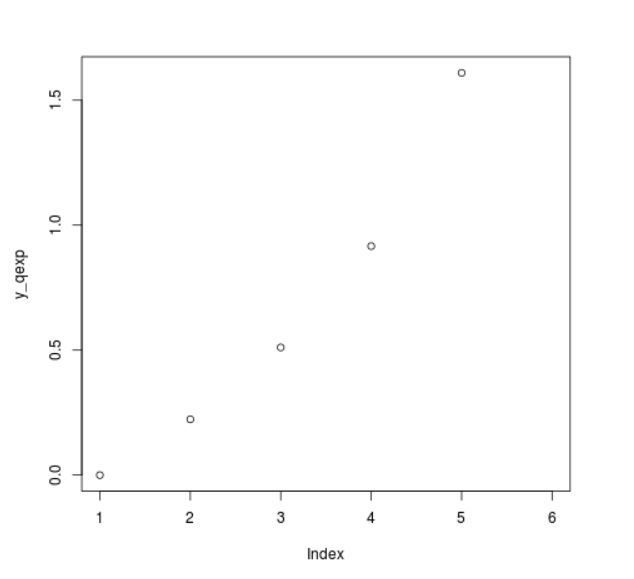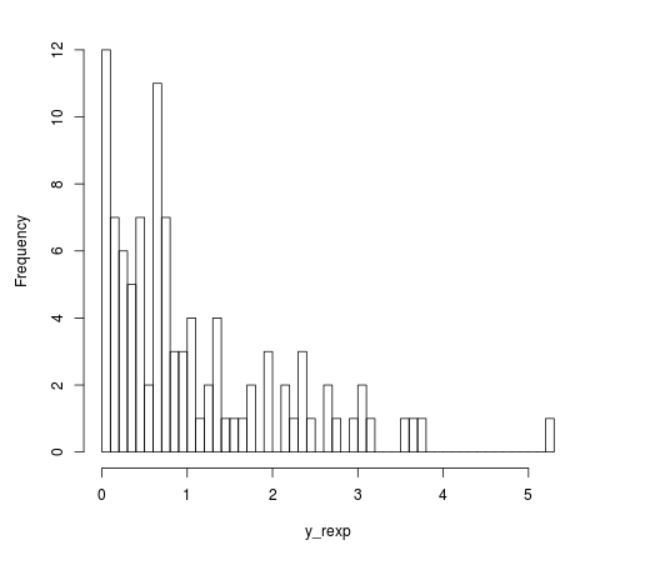Related Articles
Exponential Distribution in R Programming – dexp(), pexp(), qexp(), and rexp() Functions
• Last Updated : 08 Jul, 2020

The exponential distribution in R Language is the probability distribution of the time between events in a Poisson point process, i.e., a process in which events occur continuously and independently at a constant average rate. It is a particular case of the gamma distribution.

In R, there are 4 built-in functions to generate exponential distribution:

• dexp()
`dexp(x_dexp, rate) `
• pexp()
`pexp(x_pexp, rate )`
• qexp()
`qexp(x_qexp, rate)`
• rexp()
`rexp(N, rate )`

where,

x: represents x-values for exp function .
rate: represents the shapex.
N: Specify sample size

### Functions To Generate Exponential Distribution

#### dexp() Function

`dexp()` function returns the corresponding values of the exponential density for an input vector of quantiles.

Syntax:

`dexp(x_dexp, rate)`

Example:

 `# R program to illustrate ` `# exponential distribution ` `# Specify x-values ` `x_dexp <``-` `seq(``1``, ``10``, by ``=` `0.1``)  ` `      `  `# Apply dexp() function                ` `y_dexp <``-` `dexp(x_dexp, rate ``=` `5``)     ` `                `  `# Plot dexp values  ` `plot(y_dexp) `

Output:#### pexp() Function

`pexp()` function returns the corresponding values of the exponential cumulative distribution function for an input vector of quantiles.

Syntax:

`pexp(x_pexp, rate )`

Example:

 `# R program to illustrate ` `# exponential distribution ` ` `  `# Specify x-values ` `x_pexp <``-` `seq(``1``, ``10``, by ``=` `0.2``)                                      ` ` `  `# Apply pexp() function ` `y_pexp <``-` `pexp(x_pexp, rate ``=` `1``)  ` ` `  `# Plot values                   ` `plot(y_pexp)                                                     `

Output :#### qexp() Function

`qexp()` function gives the possibility, we can use the qexp function to return the corresponding values of the quantile function.

Syntax:

`qexp(x_qexp, rate)`

Example:

 `# R program to illustrate ` `# exponential distribution ` ` `  `# Specify x-values  ` `x_qexp <``-` `seq(``0``, ``1``, by ``=` `0.2``)                      ` `  `  `# Apply qexp() function ` `y_qexp <``-` `qexp(x_qexp, rate ``=` `1``) ` `  `  `# Plot values                    ` `plot(y_qexp)                                        `

Output:#### rexp() Function

`rexp()` function is used to simulate a set of random numbers drawn from the exponential distribution.

Syntax:

`rexp(N, rate )`

Example:

 `# R program to illustrate ` `# exponential distribution ` ` `  `# Set seed for reproducibility ` `set``.seed(``500``)  ` ` `  `# Specify size          ` `N <``-` `100` ` `  `# Draw exp distributed values ` `y_rexp <``-` `rexp(N, rate ``=` `1``) ` `  `  `# Plot exp density   ` `hist(y_rexp, breaks ``=` `50``, main ``=` `"") `

Output :My Personal Notes arrow_drop_up
Recommended Articles
Page :